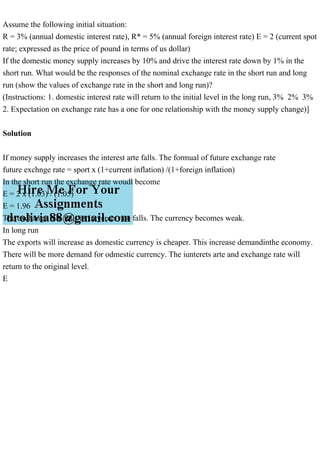Publicité

# Assume the following initial situationR = 3 (annual domestic int.pdf

25 Mar 2023Prochain SlideShareForeign Exchange Markets
Chargement dans ... 3
1 sur 1
Publicité

### Assume the following initial situationR = 3 (annual domestic int.pdf

1. Assume the following initial situation: R = 3% (annual domestic interest rate), R* = 5% (annual foreign interest rate) E = 2 (current spot rate; expressed as the price of pound in terms of us dollar) If the domestic money supply increases by 10% and drive the interest rate down by 1% in the short run. What would be the responses of the nominal exchange rate in the short run and long run (show the values of exchange rate in the short and long run)? (Instructions: 1. domestic interest rate will return to the initial level in the long run, 3% 2% 3% 2. Expectation on exchange rate has a one for one relationship with the money supply change)] Solution If money supply increases the interest arte falls. The formual of future exchange rate future exchnge rate = sport x (1+current inflation) /(1+foreign inflation) In the short run the exchange rate woudl become E = 2 x (1.03) / (1.05) E = 1.96 The exchange rate falls as intyerets rate falls. The currency becomes weak. In long run The exports will increase as domestic currency is cheaper. This increase demandinthe economy. There will be more demand for odmestic currency. The iunterets arte and exchange rate will return to the original level. E
Publicité